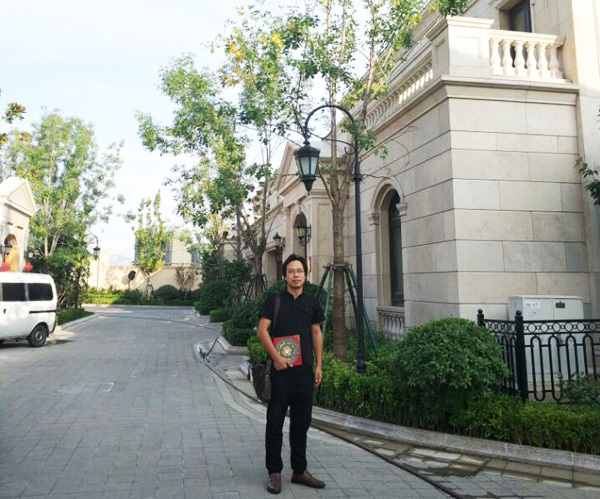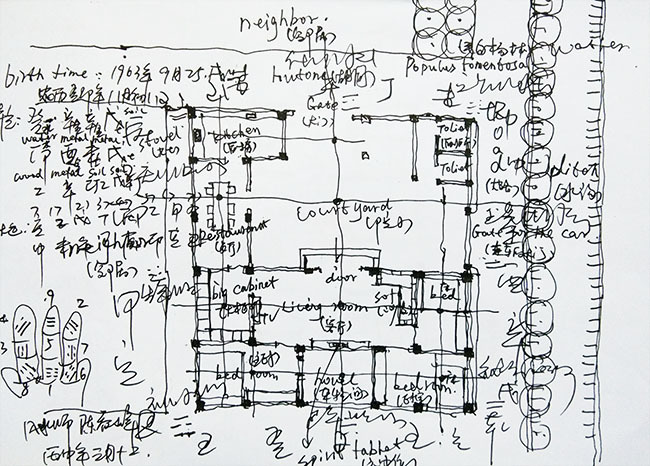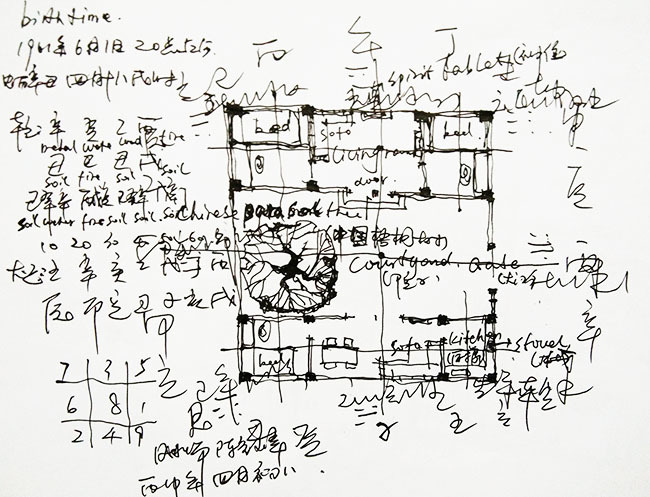|

# 院子犯五鬼不太平,陈益峰以亲身经历谈风水

风水是一个古老而新鲜的学问，以其五千年的文化历史而古老，以其不断适应当代社会的建筑形式，而不断产生新的理论，而鲜活有力。但凡有一定社会生活经历的人，尤其是在一个熟悉而稳定的社区长期居住的人，都会感受到风水的存在。陈益峰先生是一名职业的风水师傅，长期从事住宅风水、坟地风水、办公楼风水等研究。先后在北京市古建设计研究院、清华大学建筑设计研究院等单位工作，长期从事风水研究，身边发生的很多风水灾祸，让陈师傅对风水的认识更加深刻。

很多亲戚的宅子不符合风水，久住之后出现了灾祸，对陈师傅的影响非常大，也让他深刻认识到风水的作用。一个人没有风水经历，就没有风水感触。只有身边的亲戚朋友的住宅不符合风水，而又倔强地不按风水调整，这些家庭最后的结局不好，你只有从头到尾观察过这个过程，你才会深刻体会到风水的重要性。陈益峰师傅从事风水研究有15年，每天都在思考风水格局与吉凶的关系，在风水实践上面有很多有价值的体会与总结，是当代最有成就的风水师傅之一，其精湛的技艺名扬海内外，在国内外风水行业有崇高的专业威望和学术权威性，先后前往德国、波兰、澳大利亚、文莱王国、新加坡、日本、香港等地传播风水学说，影响非常广泛。

陈师傅认为人的生老病死本有定数，当泰然处之，很多人死亡属于天灾或者命数，如果房子风水再使坏，常常就更加严重了。一个人本来可以活60岁，如果风水不好，完全坑人，他可能只能活50多岁。所以风水的作用对人就是加减福气与寿命的作用。

1、案例一：捕鱼被电死

去年春天去陈师傅去他的爱人老家玩，爱人的三叔(53岁)与前面一家人的小伙子(34岁)一起用电捕鱼，一起死在河里。三叔为人聪明机灵，农村的事情搞得很透，大家很喜欢与他打交道，可惜英年早逝，可惜至极。二位家庭的宅子都犯风水五鬼（five ghosts）门(图一)，咨询陈师傅很多次，但是总觉得家里没有什么事情，至始至终就没有调整。二人的宅子都是建在村子的最西头，西边是乡村公路，南面是胡同，所以按照农村的传统规矩，将大门开在南面，做的是北房，二人家里都有车，为了走车的方便，在西面开一个大的门走车，南方离卦与西方兑卦同时开门，风水叫五鬼开门，主失火，失盗，打官司，坐牢，严重的伤人命。

另外其住宅的西边是一条南北向的河流(stream)，西南方是常年有水，西南方在后天八卦中，为坤卦，为阴。西南方在先天八卦中，为巽卦，也属阴，两个卦都是阴卦属于阴见阴为重阴之地，如果再见到阴的物质，比如住宅的西南方是河、湖、水坑、干坑、低洼地、水道、出水口。室内西南方是卫生间、大型鱼缸、缺大角、洗衣机、水龙头、浴室等，在风水不吉。

西南见水，老三（third son）影响尤其大，常常有性命之忧，其三叔（Third uncle）就是家中排行老三。还主平常多思迟疑、事业缓慢，夫妻不合，易违法、风流，孤寡辛苦、是非疾病破财，出巫婆神汉、医卜星相之人。易得胃肠病、腹疾、痔疮、肝火旺、肿瘤、增生、骨刺、结石、妇科炎症肿块、经血症等;如西南方水与南方水相连通，则主儿在父先亡;西南方水与西方水相连，主阴气重、生邪灵、怪事或阴性病;如水形呈三角形模样，则主妇女易损伤。吉凶尤其发生在老三的身上，也多发生在母亲、老母、长女，或属羊、猴之人身上;应羊、猴、牛、虎年年月。2、案例二：两个儿子都不顺

媳妇另外一个叔叔，特别有本事，家族中大小事务都是他做主，可惜家中很不太平，祸事一件接一件。大儿子结婚后，第一个孩子，生下来不久发现腿没有劲，走不了路，现在十几岁了，依然是坐轮椅。二儿子为人很正派，也很能干，可惜一次喝酒后，与村里伙伴将一个美女轮奸，坐牢十年左右。

他们家的宅子东西都是胡同，东面胡同狭小，西面胡同很宽，所以将门开在西面，做的是北房，风水犯祸害（disaster），祸害主财难积聚，官灾是非，争执被骗，，凶祸一般不大。后来儿子长大了，在院子南面盖了一个二层小楼，作为儿子结婚使用的房子，一层一个儿子。这个凶祸就大了，风水犯五鬼，南方离卦与西方兑卦互为五鬼，主失火，失盗，打官司，坐牢，严重的伤人命。

3、五鬼门路

所谓五鬼门（open the door in the Five ghosts）者最常见的是是东南巽门宅基，又有西南门往西去。西南坤门宅基，又有东南门往东行。二者皆为五鬼门。风水上也忌讳五鬼路(road in the Five ghosts)，所谓五鬼路者是东南巽门宅基，再从西南垦宫夹道内不安门往西走。西南坤门宅基又从东南巽宫夹道内不安门往东去皆为五鬼路。犯此者主贼打、火烧、官司，牵连。

五鬼在八宅风水里主火灾盗贼，口舌是非，奴仆逃走，损伤人口等。五鬼全称五鬼廉贞火，主破财连连，健康甚差，容易招鬼，游荡，恶疾，好勇斗狠，暴戾，吸毒等。五鬼指无端惹祸，但亦有转祸为福之力，宜作厕所，士多，浴室，忌作主房、大门、厨房和主气口。犯则意外多生、癌症、手术等。一般情况下农村院子常见的五鬼开门的情况有：

(1)、乾宫犯五鬼

乾宫与震宫互为五鬼，乾宫犯五鬼主损老公，当家人寿短，邪魔入侵，鬼怪病痛，官司盗贼，伤妻克子，火灾目疾，腰腿疼痛等诸凶。

(2)、坎宫犯五鬼

坎宫与艮宫互为五鬼，坎宫犯五鬼主伤中男，犯者主投河自缢，官司口舌，父子兄弟不和，腹内积块，出残疾等。

(3)、艮宫犯五鬼

坎宫与艮宫互为五鬼，艮宫犯五鬼，犯者不利小口，主投河自缢，官司口舌是非，诸事不利。

(4)、震宫犯五鬼

乾宫与震宫互为五鬼，震宫犯五鬼主子母不和，丁才不全。易得黄肿，脾胃之疾病。

(5)、巽宫犯五鬼

巽宫与坤宫互为五鬼，巽宫犯五鬼主老母长女短寿，腰腿疼痛，流产，婆媳不和，妇女性刚，不听话。

(6)、离宫犯五鬼

离宫与兑宫互为五鬼，离宫犯五鬼主伤老母，中男，少女淫乱，残疾，败坏名声。

(7)、坤宫犯五鬼

乾宫与震宫互为五鬼，坤宫犯五鬼主伤老母，长女，火灾小产，高血压，腰腿神经痛。

(8)、兑宫犯五鬼

离宫与兑宫互为五鬼，兑宫犯五鬼主淫乱夭折，妻夺夫权，男女短寿，人丁不旺，劳疾多灾，鬼神作乱。

若五鬼星与六煞或其他星连在一起，则主大凶。如走离门，艮坎位建北房，为祸害主向，坤兑位建西房，六煞连五鬼，发凶甚巨，主喝药上吊，神经不正常，主双妻。（记者/林双琪）

`声明：本文由入驻焦点开放平台的作者撰写，除焦点官方账号外，观点仅代表作者本人，不代表焦点立场错误信息举报电话： 400-099-0099，邮箱：jubao@vip.sohu.com，或点此进行意见反馈，或点此进行举报投诉。`A B C D E F G H J K L M N P Q R S T W X Y Z
A - B - C - D - E
• A
• 鞍山
• 安庆
• 安阳
• 安顺
• 安康
• 澳门
• B
• 北京
• 保定
• 包头
• 巴彦淖尔
• 本溪
• 蚌埠
• 亳州
• 滨州
• 北海
• 百色
• 巴中
• 毕节
• 保山
• 宝鸡
• 白银
• 巴州
• C
• 承德
• 沧州
• 长治
• 赤峰
• 朝阳
• 长春
• 常州
• 滁州
• 池州
• 长沙
• 常德
• 郴州
• 潮州
• 崇左
• 重庆
• 成都
• 楚雄
• 昌都
• 慈溪
• 常熟
• D
• 大同
• 大连
• 丹东
• 大庆
• 东营
• 德州
• 东莞
• 德阳
• 达州
• 大理
• 德宏
• 定西
• 儋州
• 东平
• E
• 鄂尔多斯
• 鄂州
• 恩施
F - G - H - I - J
• F
• 抚顺
• 阜新
• 阜阳
• 福州
• 抚州
• 佛山
• 防城港
• G
• 赣州
• 广州
• 桂林
• 贵港
• 广元
• 广安
• 贵阳
• 固原
• H
• 邯郸
• 衡水
• 呼和浩特
• 呼伦贝尔
• 葫芦岛
• 哈尔滨
• 黑河
• 淮安
• 杭州
• 湖州
• 合肥
• 淮南
• 淮北
• 黄山
• 菏泽
• 鹤壁
• 黄石
• 黄冈
• 衡阳
• 怀化
• 惠州
• 河源
• 贺州
• 河池
• 海口
• 红河
• 汉中
• 海东
• 怀来
• I
• J
• 晋中
• 锦州
• 吉林
• 鸡西
• 佳木斯
• 嘉兴
• 金华
• 景德镇
• 九江
• 吉安
• 济南
• 济宁
• 焦作
• 荆门
• 荆州
• 江门
• 揭阳
• 金昌
• 酒泉
• 嘉峪关
K - L - M - N - P
• K
• 开封
• 昆明
• 昆山
• L
• 廊坊
• 临汾
• 辽阳
• 连云港
• 丽水
• 六安
• 龙岩
• 莱芜
• 临沂
• 聊城
• 洛阳
• 漯河
• 娄底
• 柳州
• 来宾
• 泸州
• 乐山
• 六盘水
• 丽江
• 临沧
• 拉萨
• 林芝
• 兰州
• 陇南
• M
• 牡丹江
• 马鞍山
• 茂名
• 梅州
• 绵阳
• 眉山
• N
• 南京
• 南通
• 宁波
• 南平
• 宁德
• 南昌
• 南阳
• 南宁
• 内江
• 南充
• P
• 盘锦
• 莆田
• 平顶山
• 濮阳
• 攀枝花
• 普洱
• 平凉
Q - R - S - T - W
• Q
• 秦皇岛
• 齐齐哈尔
• 衢州
• 泉州
• 青岛
• 清远
• 钦州
• 黔南
• 曲靖
• 庆阳
• R
• 日照
• 日喀则
• S
• 石家庄
• 沈阳
• 双鸭山
• 绥化
• 上海
• 苏州
• 宿迁
• 绍兴
• 宿州
• 三明
• 上饶
• 三门峡
• 商丘
• 十堰
• 随州
• 邵阳
• 韶关
• 深圳
• 汕头
• 汕尾
• 三亚
• 三沙
• 遂宁
• 山南
• 商洛
• 石嘴山
• T
• 天津
• 唐山
• 太原
• 通辽
• 铁岭
• 泰州
• 台州
• 铜陵
• 泰安
• 铜仁
• 铜川
• 天水
• 天门
• W
• 乌海
• 乌兰察布
• 无锡
• 温州
• 芜湖
• 潍坊
• 威海
• 武汉
• 梧州
• 渭南
• 武威
• 吴忠
• 乌鲁木齐
X - Y - Z
• X
• 邢台
• 徐州
• 宣城
• 厦门
• 新乡
• 许昌
• 信阳
• 襄阳
• 孝感
• 咸宁
• 湘潭
• 湘西
• 西双版纳
• 西安
• 咸阳
• 西宁
• 仙桃
• 西昌
• Y
• 运城
• 营口
• 盐城
• 扬州
• 鹰潭
• 宜春
• 烟台
• 宜昌
• 岳阳
• 益阳
• 永州
• 阳江
• 云浮
• 玉林
• 宜宾
• 雅安
• 玉溪
• 延安
• 榆林
• 银川
• Z
• 张家口
• 镇江
• 舟山
• 漳州
• 淄博
• 枣庄
• 郑州
• 周口
• 驻马店
• 株洲
• 张家界
• 珠海
• 湛江
• 肇庆
• 中山
• 自贡
• 资阳
• 遵义
• 昭通
• 张掖
• 中卫

1室1厅1厨1卫1阳台

1
2
3
4
5

0
1
2

1

1

0
1
2
3报名成功，资料已提交审核A B C D E F G H J K L M N P Q R S T W X Y Z
A - B - C - D - E
• A
• 鞍山
• 安庆
• 安阳
• 安顺
• 安康
• 澳门
• B
• 北京
• 保定
• 包头
• 巴彦淖尔
• 本溪
• 蚌埠
• 亳州
• 滨州
• 北海
• 百色
• 巴中
• 毕节
• 保山
• 宝鸡
• 白银
• 巴州
• C
• 承德
• 沧州
• 长治
• 赤峰
• 朝阳
• 长春
• 常州
• 滁州
• 池州
• 长沙
• 常德
• 郴州
• 潮州
• 崇左
• 重庆
• 成都
• 楚雄
• 昌都
• 慈溪
• 常熟
• D
• 大同
• 大连
• 丹东
• 大庆
• 东营
• 德州
• 东莞
• 德阳
• 达州
• 大理
• 德宏
• 定西
• 儋州
• 东平
• E
• 鄂尔多斯
• 鄂州
• 恩施
F - G - H - I - J
• F
• 抚顺
• 阜新
• 阜阳
• 福州
• 抚州
• 佛山
• 防城港
• G
• 赣州
• 广州
• 桂林
• 贵港
• 广元
• 广安
• 贵阳
• 固原
• H
• 邯郸
• 衡水
• 呼和浩特
• 呼伦贝尔
• 葫芦岛
• 哈尔滨
• 黑河
• 淮安
• 杭州
• 湖州
• 合肥
• 淮南
• 淮北
• 黄山
• 菏泽
• 鹤壁
• 黄石
• 黄冈
• 衡阳
• 怀化
• 惠州
• 河源
• 贺州
• 河池
• 海口
• 红河
• 汉中
• 海东
• 怀来
• I
• J
• 晋中
• 锦州
• 吉林
• 鸡西
• 佳木斯
• 嘉兴
• 金华
• 景德镇
• 九江
• 吉安
• 济南
• 济宁
• 焦作
• 荆门
• 荆州
• 江门
• 揭阳
• 金昌
• 酒泉
• 嘉峪关
K - L - M - N - P
• K
• 开封
• 昆明
• 昆山
• L
• 廊坊
• 临汾
• 辽阳
• 连云港
• 丽水
• 六安
• 龙岩
• 莱芜
• 临沂
• 聊城
• 洛阳
• 漯河
• 娄底
• 柳州
• 来宾
• 泸州
• 乐山
• 六盘水
• 丽江
• 临沧
• 拉萨
• 林芝
• 兰州
• 陇南
• M
• 牡丹江
• 马鞍山
• 茂名
• 梅州
• 绵阳
• 眉山
• N
• 南京
• 南通
• 宁波
• 南平
• 宁德
• 南昌
• 南阳
• 南宁
• 内江
• 南充
• P
• 盘锦
• 莆田
• 平顶山
• 濮阳
• 攀枝花
• 普洱
• 平凉
Q - R - S - T - W
• Q
• 秦皇岛
• 齐齐哈尔
• 衢州
• 泉州
• 青岛
• 清远
• 钦州
• 黔南
• 曲靖
• 庆阳
• R
• 日照
• 日喀则
• S
• 石家庄
• 沈阳
• 双鸭山
• 绥化
• 上海
• 苏州
• 宿迁
• 绍兴
• 宿州
• 三明
• 上饶
• 三门峡
• 商丘
• 十堰
• 随州
• 邵阳
• 韶关
• 深圳
• 汕头
• 汕尾
• 三亚
• 三沙
• 遂宁
• 山南
• 商洛
• 石嘴山
• T
• 天津
• 唐山
• 太原
• 通辽
• 铁岭
• 泰州
• 台州
• 铜陵
• 泰安
• 铜仁
• 铜川
• 天水
• 天门
• W
• 乌海
• 乌兰察布
• 无锡
• 温州
• 芜湖
• 潍坊
• 威海
• 武汉
• 梧州
• 渭南
• 武威
• 吴忠
• 乌鲁木齐
X - Y - Z
• X
• 邢台
• 徐州
• 宣城
• 厦门
• 新乡
• 许昌
• 信阳
• 襄阳
• 孝感
• 咸宁
• 湘潭
• 湘西
• 西双版纳
• 西安
• 咸阳
• 西宁
• 仙桃
• 西昌
• Y
• 运城
• 营口
• 盐城
• 扬州
• 鹰潭
• 宜春
• 烟台
• 宜昌
• 岳阳
• 益阳
• 永州
• 阳江
• 云浮
• 玉林
• 宜宾
• 雅安
• 玉溪
• 延安
• 榆林
• 银川
• Z
• 张家口
• 镇江
• 舟山
• 漳州
• 淄博
• 枣庄
• 郑州
• 周口
• 驻马店
• 株洲
• 张家界
• 珠海
• 湛江
• 肇庆
• 中山
• 自贡
• 资阳
• 遵义
• 昭通
• 张掖
• 中卫• 手机• 分享
• 设计
免费设计
• 计算器
装修计算器
• 入驻
合作入驻
• 联系
联系我们
• 置顶
返回顶部